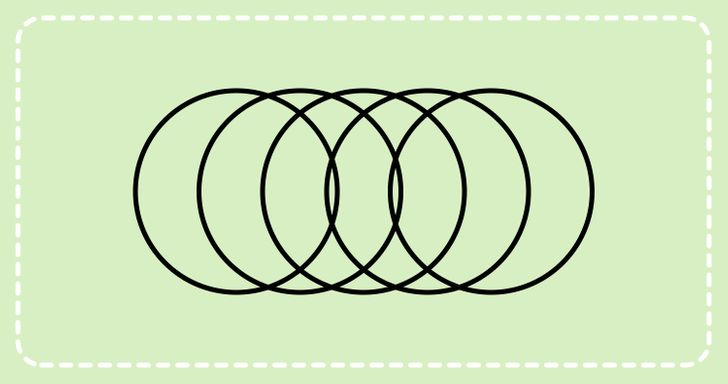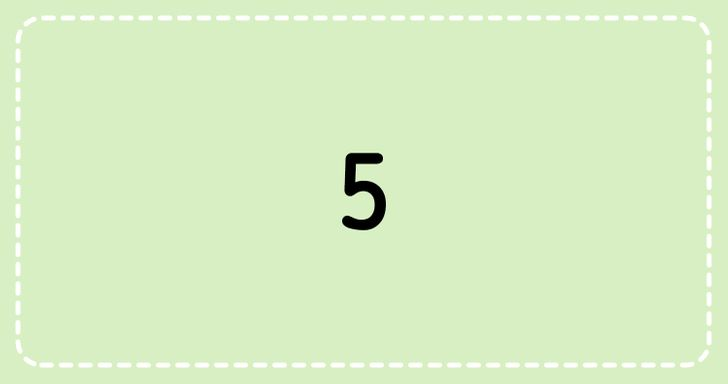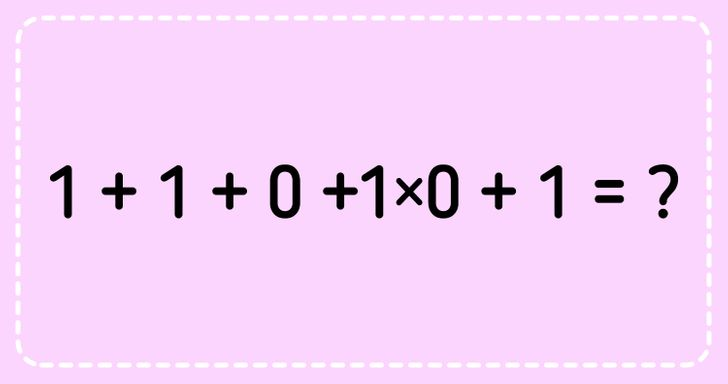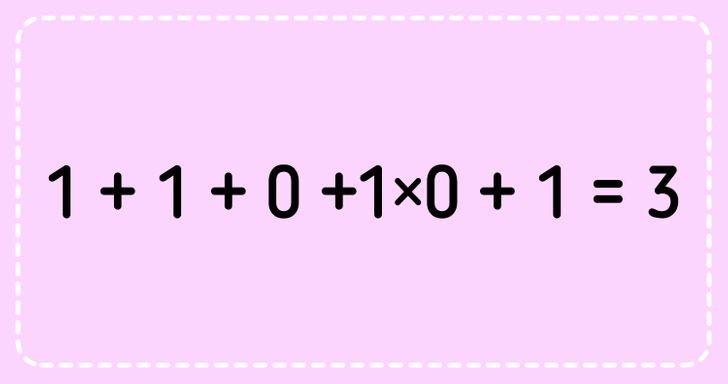# 15 Brain Teasers That Will Instantly Boost Your Mental Power

Math can look confusing and terrifying at first glance. But under the rough surface lies a beautiful world of wonder, exploration, and joy. Arithmetic can even promote your cognitive function and sharpen your critical thinking. Yep, even in the leisure of your own home.

So are you looking for an entertaining but also challenging activity? We at Bright Side are here to deliver 15 math puzzles to you that will boost your mood and mental power better than your morning coffee.

### 1. What is the value of the equation?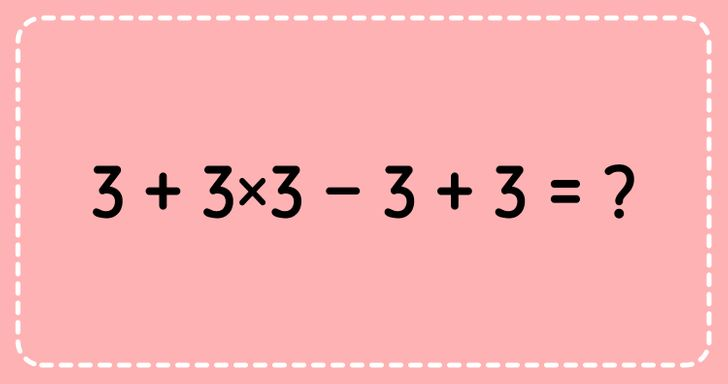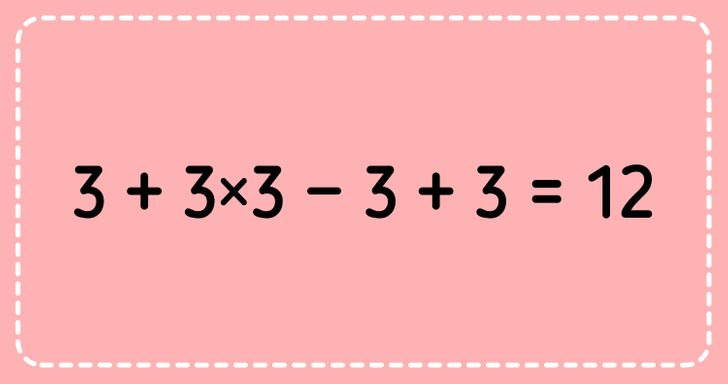### 2. What digit is N so the equation is correct?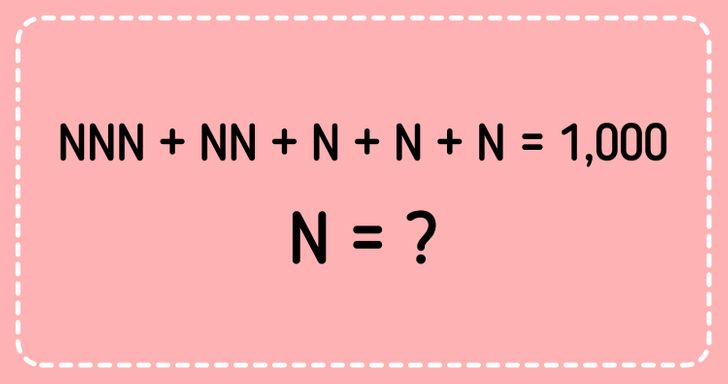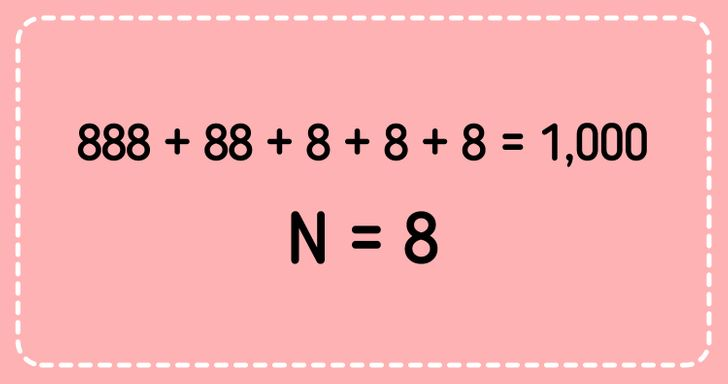### 3. Use: “+‘, ’-”, “x”, “/”. Add the appropriate one between the digits of the numbers in order to make the equation right.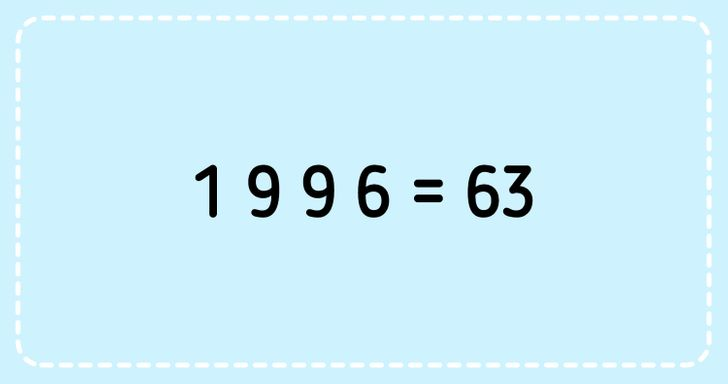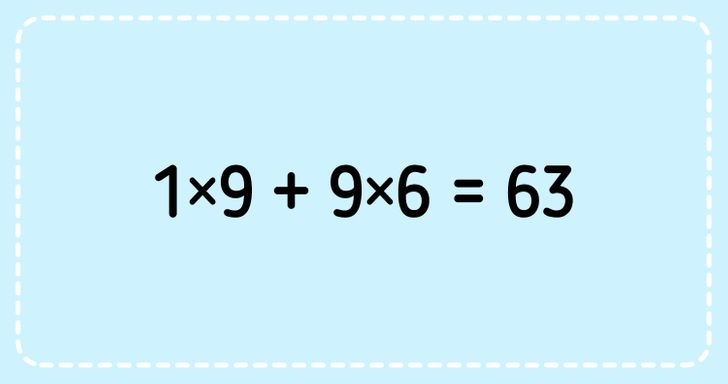### 4. How many triangles are in the picture?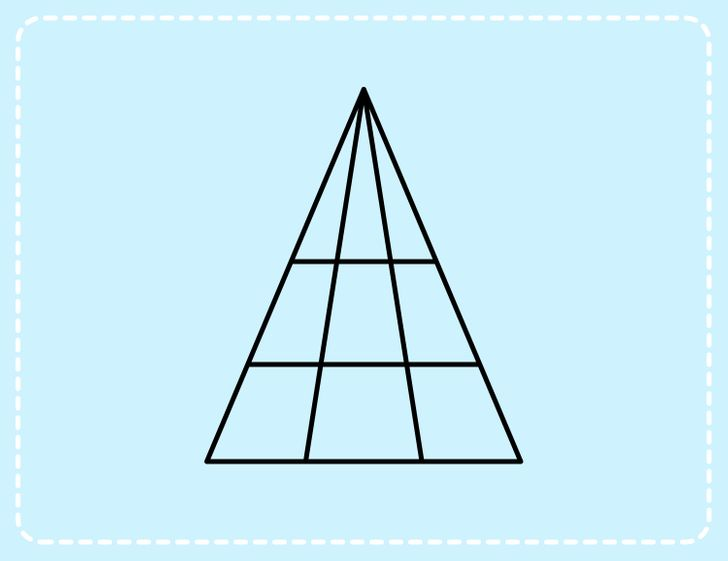### 5. How much does one ball weigh?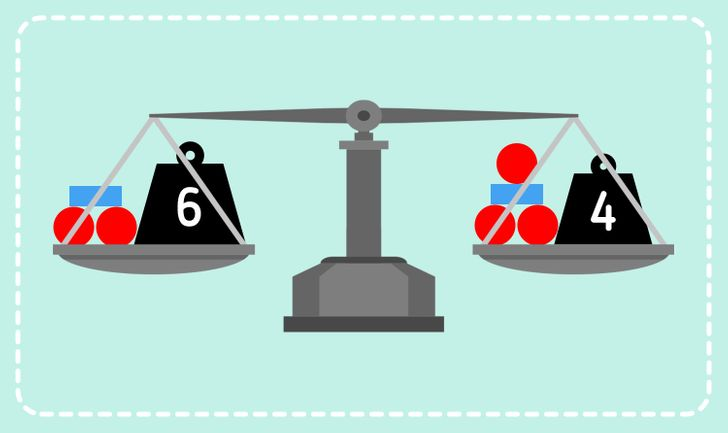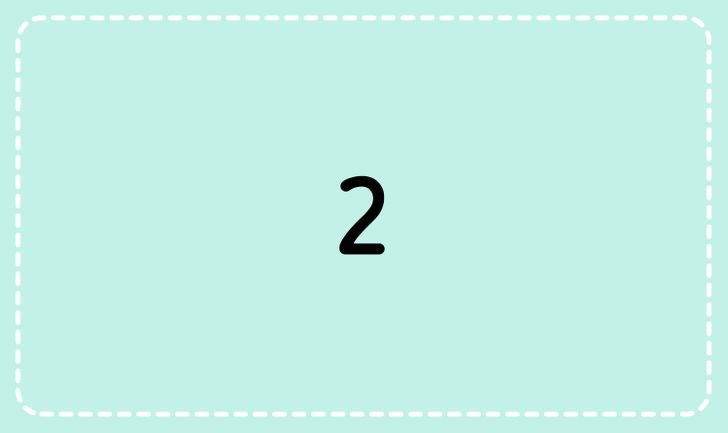### 6. Replace the letters with digits in order to make the equation right.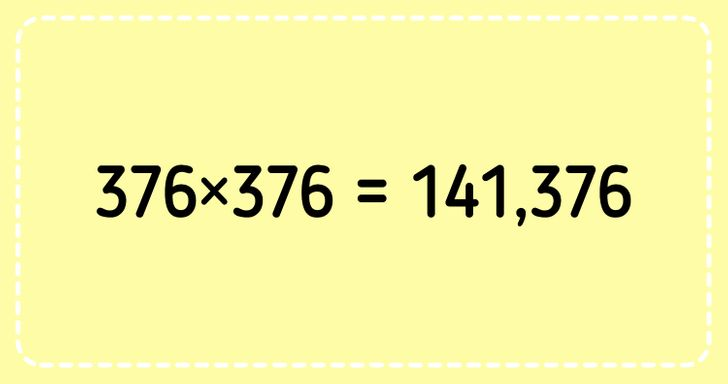### 7. Find the values.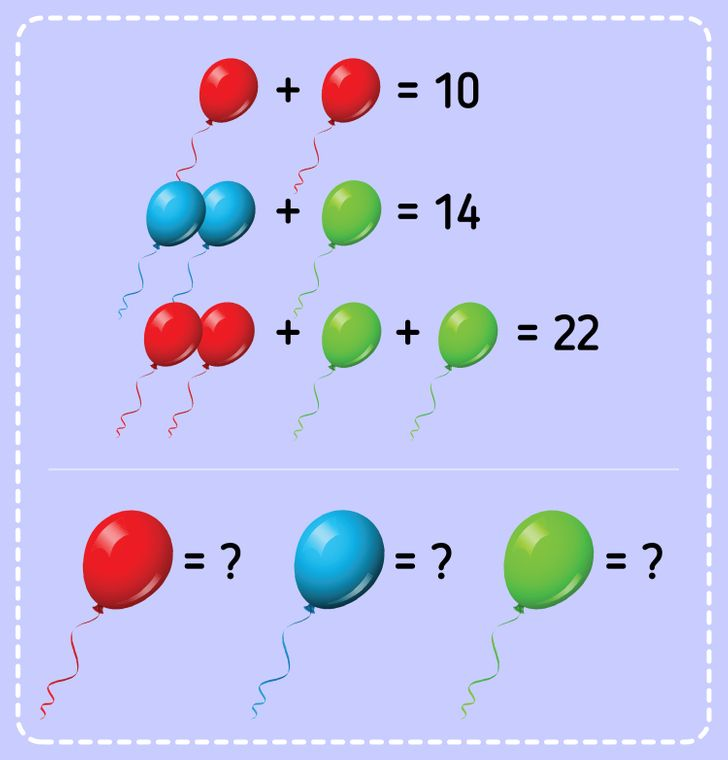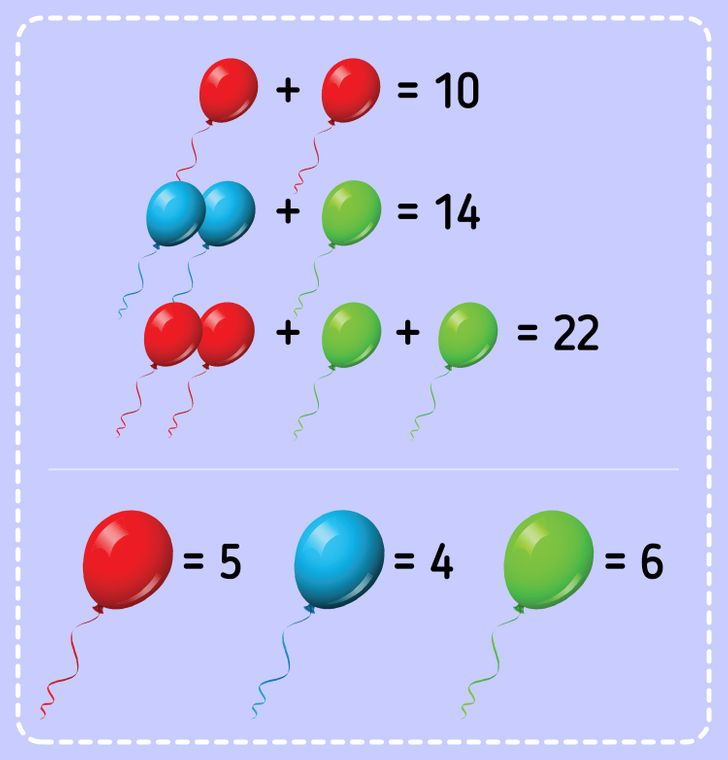### 8. How many balls do you have to use to balance the second scale?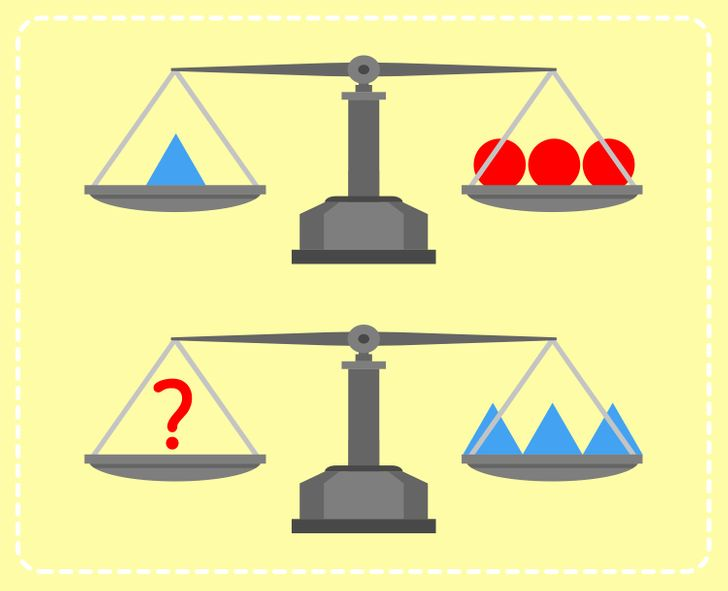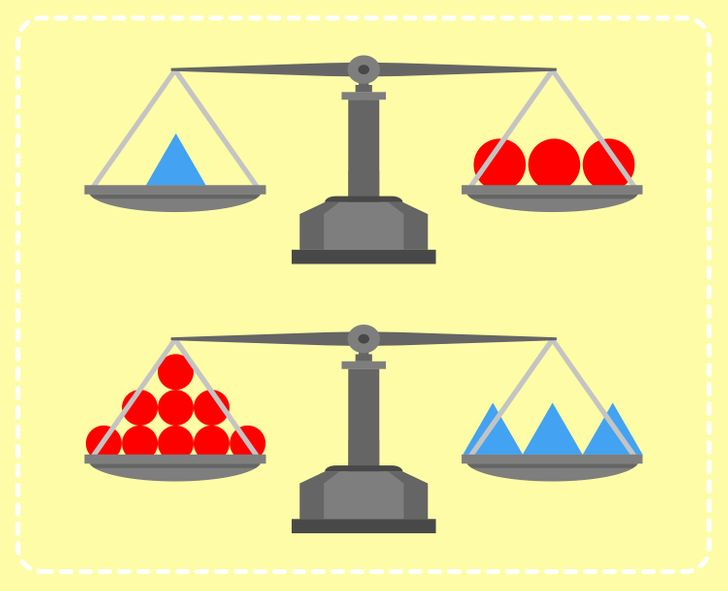### 9. Use digits from 0 to 9. The sum of the 3 values on each side of the triangle must be 9.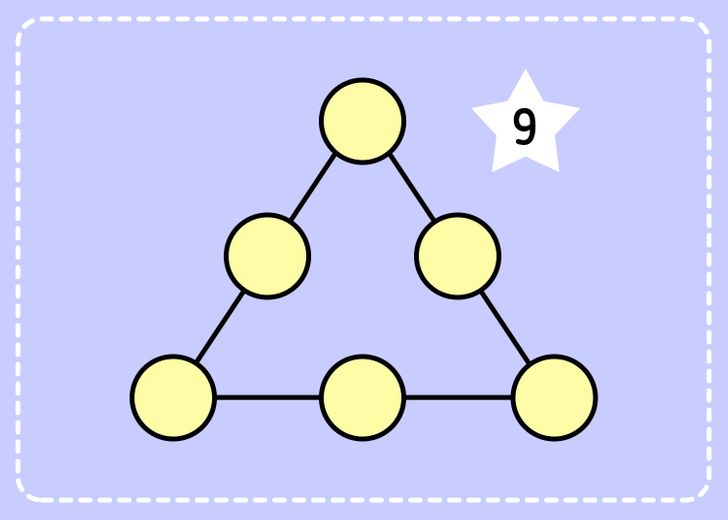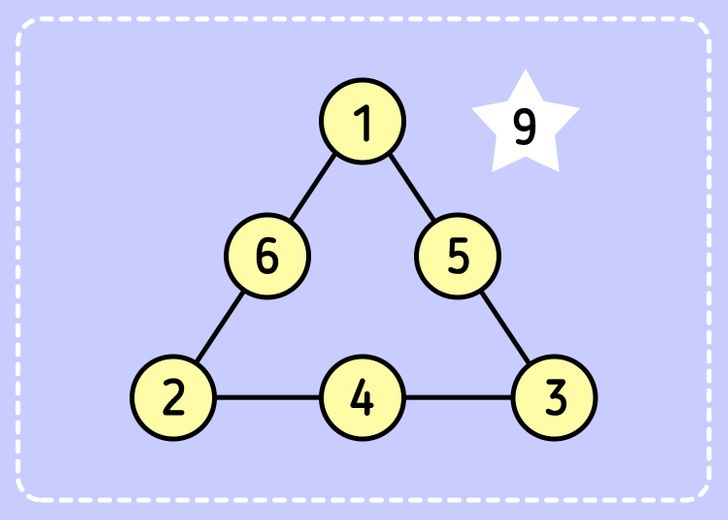### 10. Replace the letters with digits in order to make the equation right.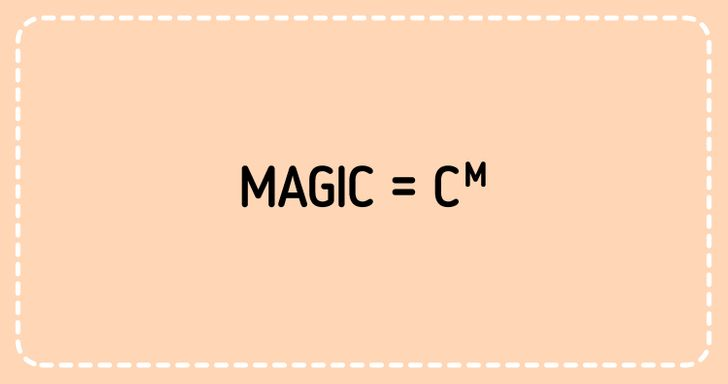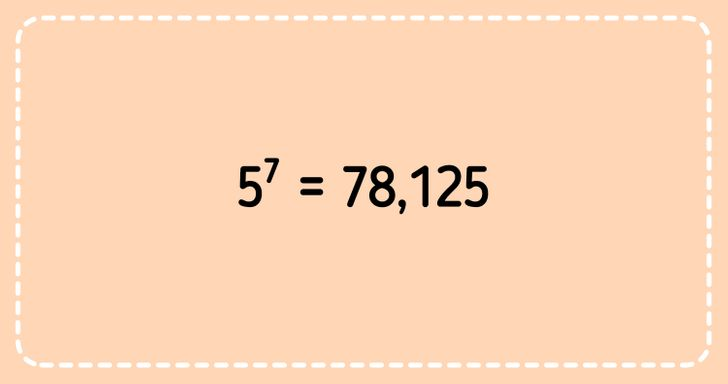### 11. What is the next number in the series?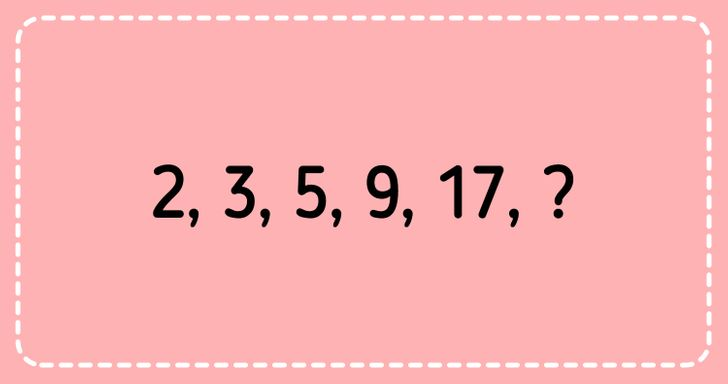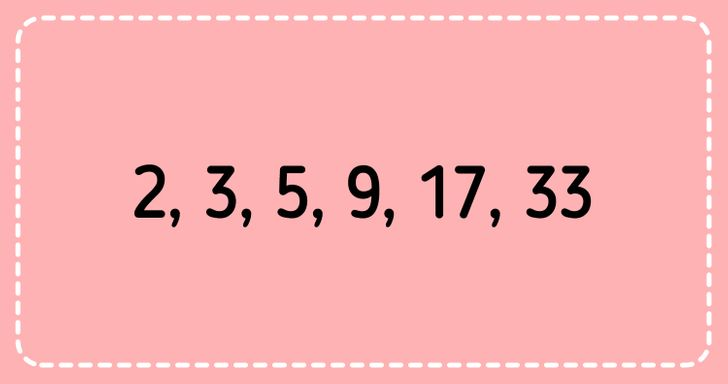### 12. Move 3 matches to create 4 rectangles.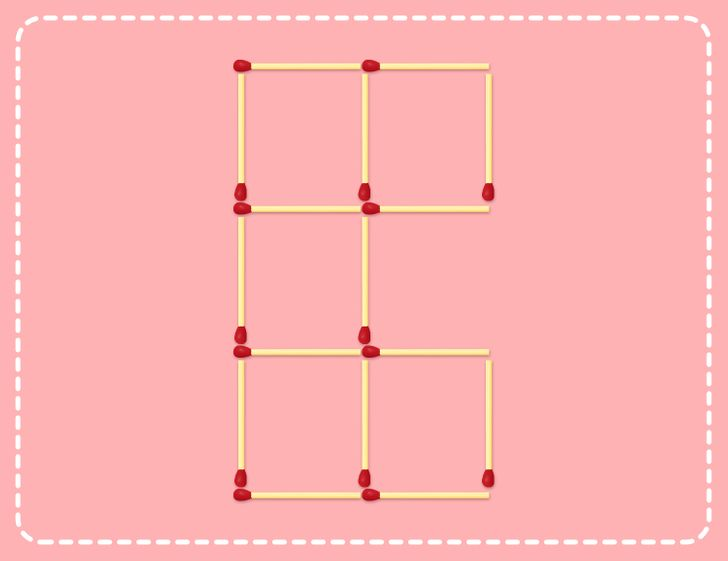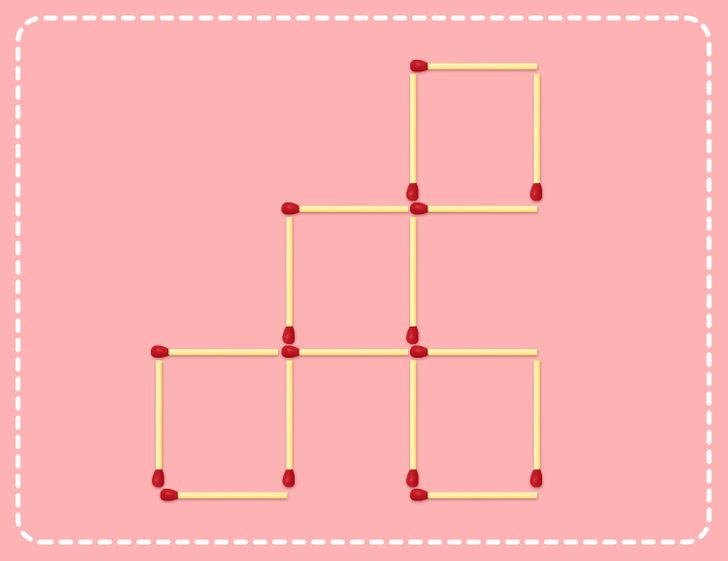### 13. Crack the code using the instructions.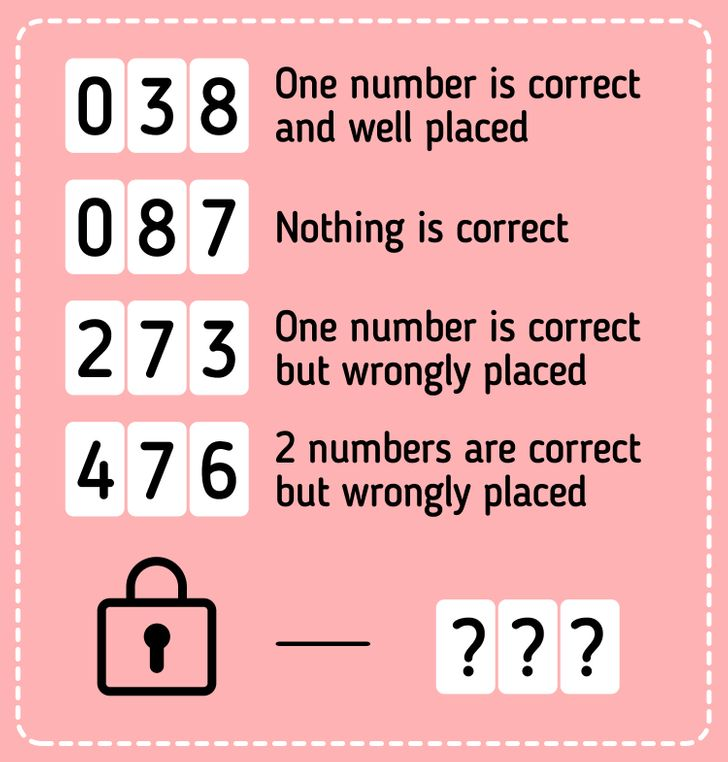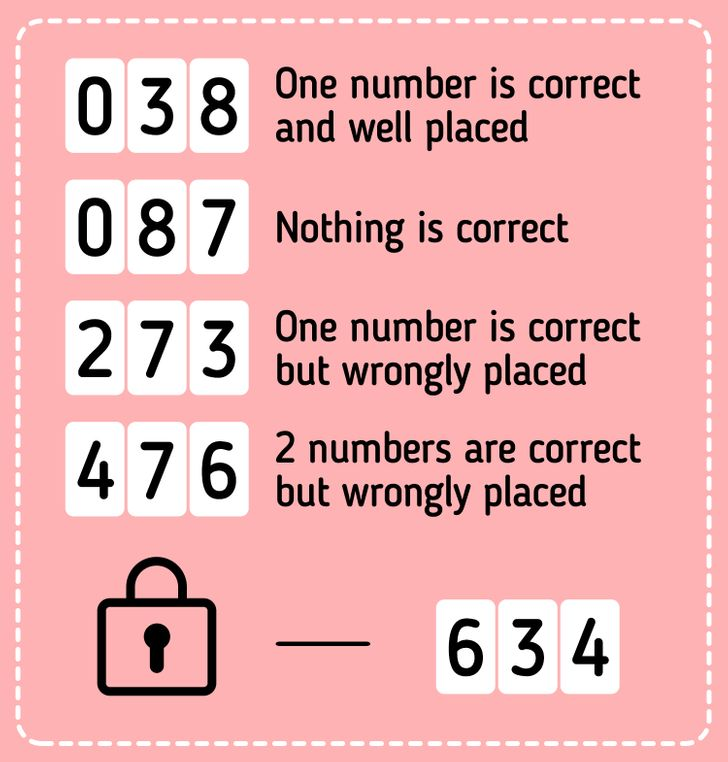### 14. How many circles are in the picture?